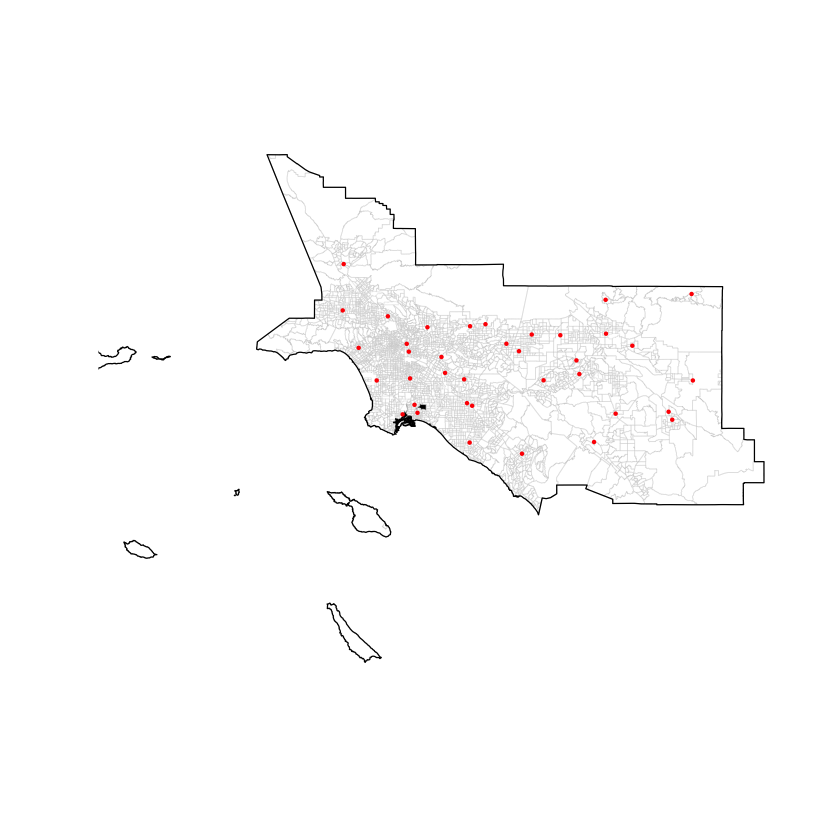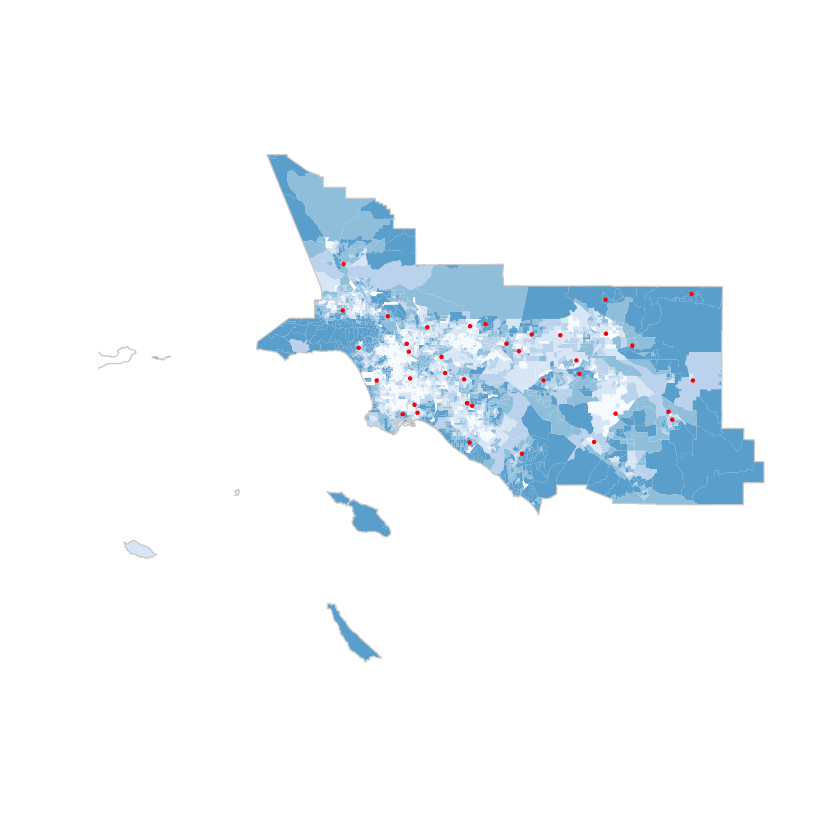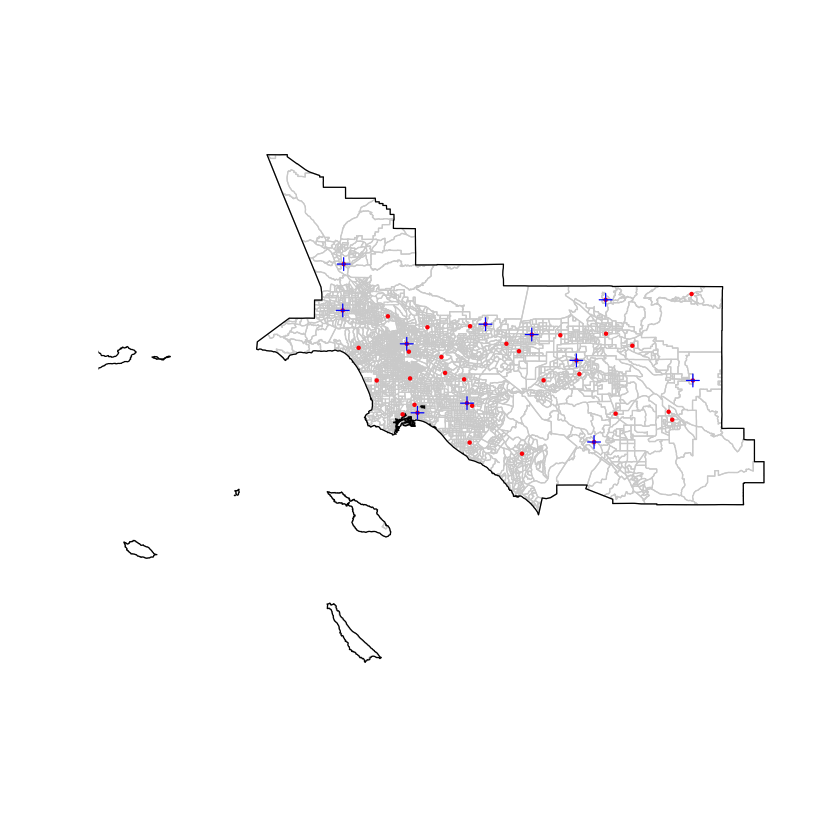## Spatial Analysis¶

Research in R for a project looking into the correlation between pollution (levels of PM2.5) and race (levels of diversity) in Los Angeles. This notebook contains code used to calculate spatial and statistical measures and to plot diagrams and figures for the resulting paper 'The effect of exposure to particulate matter on the racial diveristy of populations across Los Angeles'.

### Package Installation¶

In [ ]:
install.packages(c('rgeos','rgdal','RColorBrewer','GISTools','raster','gstat','nlme','sp','geosphere','moments','plyr'))

In :
options(warn=-1)
library(rgeos)
library(rgdal)
library(RColorBrewer)
library(GISTools)
library(raster)
library(gstat)
library(nlme)
library(sp)
require(geosphere)
library(spdep)
library(spgwr)
library(GWmodel)
library(moments)
library(plotrix)
library(spatstat)
library(maptools)
library(plyr)


### Prep Pollution Data¶

Data has been collected from the California Environmental Protection Agency - these measures of particulate matter were taken from a total of 37 monitoring stations in the South Coast Air Basin, providing an hourly average each day throughout the month of April 2016.

In :
#read pollution data

#read shapefiles of EPA monitoring stations and the air basins

#extract points within the South coast
SC.monitor = monitor[monitor$AIRBASIN %in% c("South Coast"),] SC.AirBasin = Ca.AirBasin[Ca.AirBasin$NAME %in% c("South Coast"),]

#reproject the data to a suitable projection (utm used here because of the scale of the analysis)
SC.monitor.t = spTransform(SC.monitor, CRS("+proj=longlat +datum=NAD83 +no_defs +ellps=GRS80 + towgs84=0,0,0"))
SC.AirBasin.t = spTransform(SC.AirBasin, CRS("+proj=longlat +datum=NAD83 +no_defs +ellps=GRS80 +towgs84=0,0,0"))

OGR data source with driver: ESRI Shapefile
Source: "data/airmonitoringstations.shp", layer: "airmonitoringstations"
with 296 features
It has 12 fields
OGR data source with driver: ESRI Shapefile
Source: "data/CaAirBasin.shp", layer: "CaAirBasin"
with 15 features
It has 5 fields
Integer64 fields read as strings:  CAABA_ CAABA_ID


### Prep Census Data¶

The census data was sourced from the American Community Survey. These frequencies of different races in census tracts throughout Los Angeles County are 5-year estimates based on Census 2010 data, with a 90% margin of error. A new measure of diversity is calculated which is a percentage measure of those in the population who are not in the majority race.

In :
#read california census tracts
all.tracts = shapefile("data/cb_2015_06_tract_500k.shp")

#reproject the data to match the projection of the pollution datasets (utm)
all.tracts.t = spTransform(all.tracts, CRS("+proj=longlat +datum=NAD83 +no_defs + ellps=GRS80 +towgs84=0,0,0"))

#extract census tracts that intersect with the sc air basin
SC.tracts.t <- intersect(all.tracts.t, SC.AirBasin.t)

#read in the census data and merge with census tracts

#race data set up
race.data = census.data[,c("GEO.id", "HD01_VD01", "HD01_VD02","HD01_VD03", "HD01_VD04","HD01_VD05","HD01_VD06")]
colnames(race.data)[1:7] = c("AFFGEOID","Total","White","Black","AIndian","Asian","Hawaiian")
#delete unneccessary info
race.data <- race.data[c(-1),]

#function for getting diversity measure
getdiversity <- function(rowval) {
n = rowval
tempmax = max(as.numeric(as.character(race.data$White[n])),as.numeric(as.character(race.data$Black[n])),as.numeric(as.character(race.data$AIndian[n])),as.numeric(as.character(race.data$Asian[n])),as.numeric(as.character(race.data$Hawaiian[n]))) diversity = tempmax / as.numeric(as.character(race.data$Total[n]))
return(diversity)
}

Diversity <- matrix(, nrow=nrow(race.data))
for (i in 1:nrow(race.data)) {
Diversity[i] = getdiversity(i)
}
race.data = cbind(race.data, Diversity)

#income data set up
income.data = census2.data[,c("GEO.id", "HD01_VD01")]
colnames(income.data)[1:2] = c("AFFGEOID","Income")

#change the name of GEO.id so it can be merged with shapefile
colnames(census.data) = "AFFGEOID"

#merge the sc census tracts with the census data
SC.tracts.t = sp::merge(SC.tracts.t, race.data, by='AFFGEOID')
SC.tracts.t = sp::merge(SC.tracts.t, income.data, by='AFFGEOID')

#remove missing values
SC.tracts.t <- SC.tracts.t[complete.cases(SC.tracts.t$Diversity),]  ### Air Basins of LA¶ First let's check out where our South Coast air basins are in LA, and we can project them on top of a choropleth map of our new diversity measure too to see if that is telling us anything interesting. In : #map the south coast air basin with census tracts and monitoring station locations par(mfrow=c(1,1)) plot(SC.tracts.t, border="lightgrey", lwd=0.3, col=SC.tracts.t$Diversity)In :
#choropleth map of diversity values
shades = auto.shading(as.numeric(SC.tracts.t$Diversity),cols=brewer.pal(9,"Blues")) choropleth(SC.tracts.t,SC.tracts.t$Diversity,shading=shades, border=NA)### Ozone Data¶

We can calculate some average ozone recordings for these sites

In :
#calculate mean and max ozone level for each site for all readings
mean.partmat <- aggregate(value ~ site, partmat, mean)
max.partmat <- aggregate(value ~ site, partmat, max)

#join the mean and max ozone values to their respective monitoring stations
#and rename the first col of monitoring data to site for merging purposes
names(SC.monitor.t) <- "site"

#merge SC.monitor with ozone mean and max data
mrg.tab.mean <- sp::merge(SC.monitor.t, mean.partmat, by="site", all.x=FALSE)
mrg.tab.max <- sp::merge(SC.monitor.t, max.partmat, by="site", all.x=FALSE)

#create a max and a mean spatialPointDataFrame from data
partmat.mean.spdf = na.omit(mrg.tab.mean)
partmat.max.spdf = na.omit(mrg.tab.max)

In :
#add the merged moniotring stations to map in blue (there are less stations now as a result of merge)
par(mfrow=c(1,1))
plot(SC.tracts.t, border="lightgrey", col=SC.tracts.t$Diversity) plot(SC.AirBasin.t, add=T) plot(SC.monitor.t, add=T, col="red", cex=0.5, pch=16) plot(partmat.mean.spdf,add=T,col="blue")### Correlation and Regression Analysis¶ In : head(SC.tracts.t)  AFFGEOIDSTATEFPCOUNTYFPTRACTCEGEOIDNAME.1LSADALANDAWATERAREACAABA_IDNAME.2TotalWhiteBlackAIndianAsianHawaiianDiversityIncome 121400000US0603710210506 037 102105 06037102105 1021.05 CT 492761 0 18037550502 18 South Coast 1880 1249 37 5 131 0 0.6643617 46429 311400000US0603710450006 037 104500 06037104500 1045 CT 531002 0 18037550502 18 South Coast 2906 2311 0 0 5 0 0.7952512 49375 391400000US0603710482206 037 104822 06037104822 1048.22 CT 604352 0 18037550502 18 South Coast 2228 1533 5 23 156 0 0.6880610 42684 561400000US0603710664206 037 106642 06037106642 1066.42 CT 1577594 0 18037550502 18 South Coast 3323 2456 48 0 290 0 0.7390912 82159 751400000US0603710960106 037 109601 06037109601 1096.01 CT 2214559 33284 18037550502 18 South Coast 5285 3085 113 51 771 0 0.5837275 73083 801400000US0603711110006 037 111100 06037111100 1111 CT 1059245 0 18037550502 18 South Coast 3017 1670 222 0 464 0 0.5535300 72625 In : DV = as.numeric(SC.tracts.t$White)
INDV = as.numeric(SC.tracts.t$Black) #plot(DV,INDV) cor.test(DV,INDV) #first, plot data and fit linear line through it based on least squares #lmfit = lm(DV~INDV) #abline(lmfit) #conduct regression analysis linear.model = lm(DV~INDV) summary(linear.model) resids = residuals(linear.model)   Pearson's product-moment correlation data: DV and INDV t = 0.81147, df = 3478, p-value = 0.4172 alternative hypothesis: true correlation is not equal to 0 95 percent confidence interval: -0.01947720 0.04696343 sample estimates: cor 0.0137583  Call: lm(formula = DV ~ INDV) Residuals: Min 1Q Median 3Q Max -1807.6 -930.2 -167.5 798.0 2463.9 Coefficients: Estimate Std. Error t value Pr(>|t|) (Intercept) 1.765e+03 3.587e+01 49.211 <2e-16 *** INDV 4.170e-02 5.139e-02 0.811 0.417 --- Signif. codes: 0 ‘***’ 0.001 ‘**’ 0.01 ‘*’ 0.05 ‘.’ 0.1 ‘ ’ 1 Residual standard error: 1131 on 3478 degrees of freedom Multiple R-squared: 0.0001893, Adjusted R-squared: -9.818e-05 F-statistic: 0.6585 on 1 and 3478 DF, p-value: 0.4172  In : #prepare data for plotting residuals from the regression analysis #first convert census tracts to a data frame object (need to not use spdf for now) tracts.df = as.data.frame(SC.tracts.t) #combine census data with residuals from census data tracts.df = cbind(tracts.df,resids) #change col header name colnames(tracts.df) = "residuals" #merge the residuals data set with the census data set SC.tracts.t = merge(SC.tracts.t, tracts.df, by='AFFGEOID')  In : #set min and max lat/long for mapping residuals minlat = min(SC.monitor$LATITUDE - 0.1)
maxlat = max(SC.monitor$LATITUDE + 0.1) minlong = min(SC.monitor$LONGITUDE - 0.1)
maxlong = max(SC.monitor$LONGITUDE + 0.1) #create a colouring scheme for choropleth map shades = auto.shading(as.numeric(SC.tracts.t$residuals),cols=brewer.pal(9,"Greens"))

#create a choropleth map